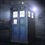# $A\ very\ simple,yet\ elegant\ NT\ proof---II$

$This\ is\ the\ second\ note\ on\ simple\ NT\ proofs.\\ This\ proof\ is\ about\ the\ Euclidean\ Algorithm\ which\ helps\ us\ to\ calculate\ the\\\ Greatest\ Common\ Divisor\ of\ humongous\ numbers.\\This\ proof\ is\ super\ simple\ but\ I\ bet\ you\ will\ like\ it.\\Let\ us\ take\ two\ positive\ integers\\ 'a'\ and\ 'b' and\ calculate\ their\ gcd.$ $The\ Euclidean\ algorithm\ states\ that:gcd(a,b)=gcd(a-b,b)\ if\ a>b.$ $Let\ us\ say\ that\ the\ gcd(a,b)=x\\ \Longrightarrow\ a=x*p\ and\ b=x*q.$ $Statement\ 1:$$gcd(p,q)=1$i.e they are co-prime. $Proof\ of\ statement\ 1:\$/extract_itex]We will prove this by contradiction.Let us say that $gcd(p,q)=r\ \Longrightarrow\ p=r*y\ and\ q=r*z$$\Longrightarrow\ a=x*r*y\ and\ b=x*r*z$$\Longrightarrow\ gcd(a,b)=x*r,$which is a contradiction.Thus,$'p'$ and$'q'$ are co-prime. $Statement\ 2:gcd(a.b)=gcd(a-b,b)$We know that $a=x*p\ and\ b=x*q,substituting\ in\ the\ equation\ gives\\\ x=gcd(x(p-q),x(q)).$$This\ can\ be\ easily\ done\ if\ we\ prove\ that \ gcd((p-q),(q))=1.$$We\ do\ this\ by\ contradiction.\\Let\ us\ say\ that\ gcd((p-q),q)=t\\\Longrightarrow\ (p-q)=t*m\ and\ q=t*n\\\ \Longrightarrow\ p-(t*n)=t*m\ \Longrightarrow\ p=t*(m+n)\ and\ from\ above\ we\ have\\:q=t*n.But,this\ can't\ happen\ as\ gcd(p,q)=1.$$Thus,gcd((p-q)(q)=1$$PROVED!!$ $P.S:This\ isn't\ a\ copied\ proof.$Note by Adarsh Kumar 6 years, 9 months ago This discussion board is a place to discuss our Daily Challenges and the math and science related to those challenges. Explanations are more than just a solution — they should explain the steps and thinking strategies that you used to obtain the solution. Comments should further the discussion of math and science. When posting on Brilliant: • Use the emojis to react to an explanation, whether you're congratulating a job well done , or just really confused . • Ask specific questions about the challenge or the steps in somebody's explanation. Well-posed questions can add a lot to the discussion, but posting "I don't understand!" doesn't help anyone. • Try to contribute something new to the discussion, whether it is an extension, generalization or other idea related to the challenge. • Stay on topic — we're all here to learn more about math and science, not to hear about your favorite get-rich-quick scheme or current world events. MarkdownAppears as *italics* or _italics_ italics **bold** or __bold__ bold - bulleted- list • bulleted • list 1. numbered2. list 1. numbered 2. list Note: you must add a full line of space before and after lists for them to show up correctly paragraph 1paragraph 2 paragraph 1 paragraph 2 [example link](https://brilliant.org)example link > This is a quote This is a quote  # I indented these lines # 4 spaces, and now they show # up as a code block. print "hello world" # I indented these lines # 4 spaces, and now they show # up as a code block. print "hello world" MathAppears as Remember to wrap math in $$ ... $$ or \[ ... $ to ensure proper formatting.
2 \times 3 $2 \times 3$
2^{34} $2^{34}$
a_{i-1} $a_{i-1}$
\frac{2}{3} $\frac{2}{3}$
\sqrt{2} $\sqrt{2}$
\sum_{i=1}^3 $\sum_{i=1}^3$
\sin \theta $\sin \theta$
\boxed{123} $\boxed{123}$

Sort by: